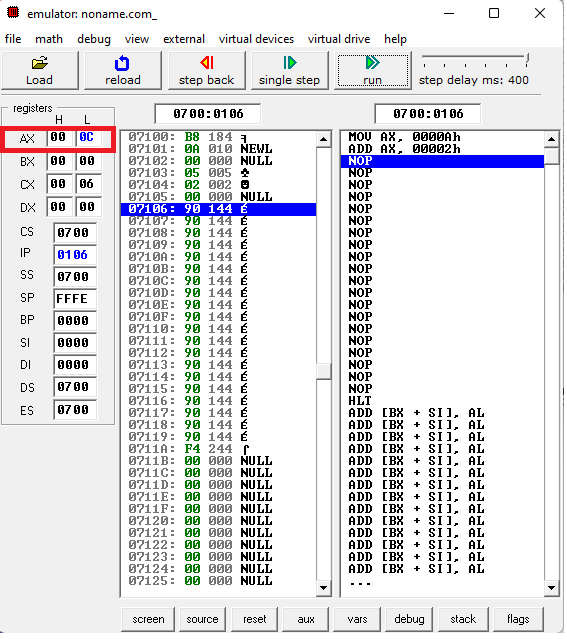﻿ How to Perform Addition, Subtraction, Multiplication, and Division in x86 Assembly Language ﻿# How to Perform Addition, Subtraction, Multiplication, and Division in x86 Assembly LanguageIn this article, we show how to perform the arithmetic operations of addition, subtraction, multiplication, and division in x86 assembly language.

These are the most basic mathematical operations.

Ax is the accumulator register. It is the register used most commonly when performing arithmetic operations.

So when we are adding or subtracting numbers, we do this all in the accumulator (ax) register.

If you are working with small numbers, you can use the al register, which is the lower 8 bits of the ax register. This allows you to work with bits up to 256 (28).

Other than that, you can just work with the complete 16-bit ax register.

Multiplication and division are a little different in that you use the ax register but you must also use another register, the bx register, in order to actually perform the multiplication operation.

So let's start going over the arithmetic operations.

This is shown below.

So addition is the sum of at least 2 numbers.

How it works in assembly is that we move the first number to the ax register, again, the accumulator register. In this case, this is 10.

Now we want to add a number to this 10, which is in the accumalator register, ax.

We do this with the add operation.

10 + 2 is 12 in decimal or 0C in hexadecimal.

Get used to hexadecimal when working with x86 assembly, because that's the output you'll always see in arithmetic operations. The output will be in hexadecimal.

After running this code, at the end, you should see that there is now 0C in the AX register.

This is shown below.If you want to add more, you can run the add operations however many times you need.

This is shown in the code below.

The program above produces the output of 0D in hexadecimal, or 13 in decimal.

### Subraction in x86

So now we move on to subtraction, which is very similar to addition.

The sub operation allows us to subtract in x86 assembly.

This is shown below.

So we first move 10 into the accumulator register.

We then use the sub operation to subtract 2 from what's the in the AX register, which is 10.

This leaves us with an output of 8.

### Multiplication in x86

So now we move on to multiplication and division, which is different than addition or subtraction.

Unlike addition and subtraction, multiplication and division require an additional register other than the AX register.

We place one operand in the AX register.

We place the other operand in the BX register.

We then use the mul operation on the BX register, which will then multiply the 2 operands and store the result in the AX register.

Below we multiply 10 and 2 and get an output of 14 in hex or 20 in decimal.

So we first move 10 into the accumulator register.

We then move 2 into the BX register.

We now have our 2 operands that we want to multiply.

In order to multiply them, we use the mul operation on the BX register, which does the multiplication of the 2 numbers and stores the result in the AX register.

This is how multiplication is done.

If you want to multiply more than one number, then you perform the multiplication and then move the next number into the BX register after performing the first multiplication operation, and then use the mul operation again on the BX register.

This is shown in the code below.

In this example, we first multiply 2 and 10 to get 14 hex or 20 decimal.

We then want to multiply this product by 4. So we move in 4 into the BX register after the initial multiplication. We then do the mul operation again on the BX register. As output, we get 50 in hexadecimal or 80 in decimal.

So this is how we can multiple multiplication operations in x86 assembly language.

### Division in x86

So now we move on to division, which is similar to multiplication.

We place one operand, the quotient, in the AX register.

We place the other operand, the divisor, in the BX register.

We then use the div operation on the BX register, which will divide the quotient by the divisor and store the result in the AX register.

Below we divide 10 by 2 and get an output of 5.

So we first move 10 into the accumulator register.

We then move 2 into the BX register.

We now have our 2 operands that we want to divide.

In order to perform division, we use the div operation on the BX register, which does the division of the 2 numbers and stores the result in the AX register.

This is how division is done.

This is how we perform the arithmetic operations of addition, subtraction, multiplication, and division in the x86 assembly programming language.

Related Resources